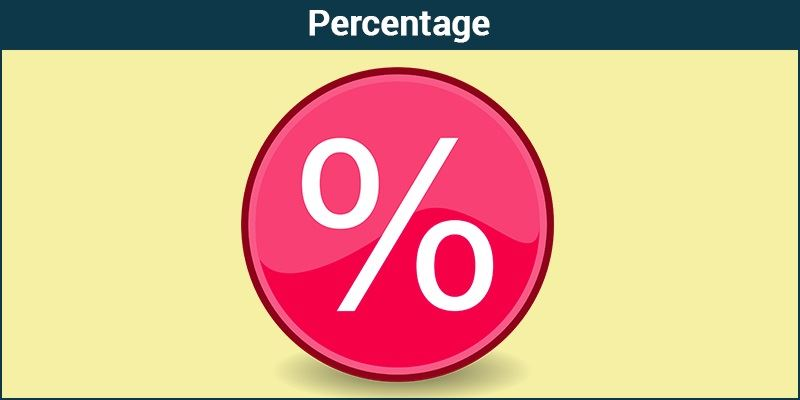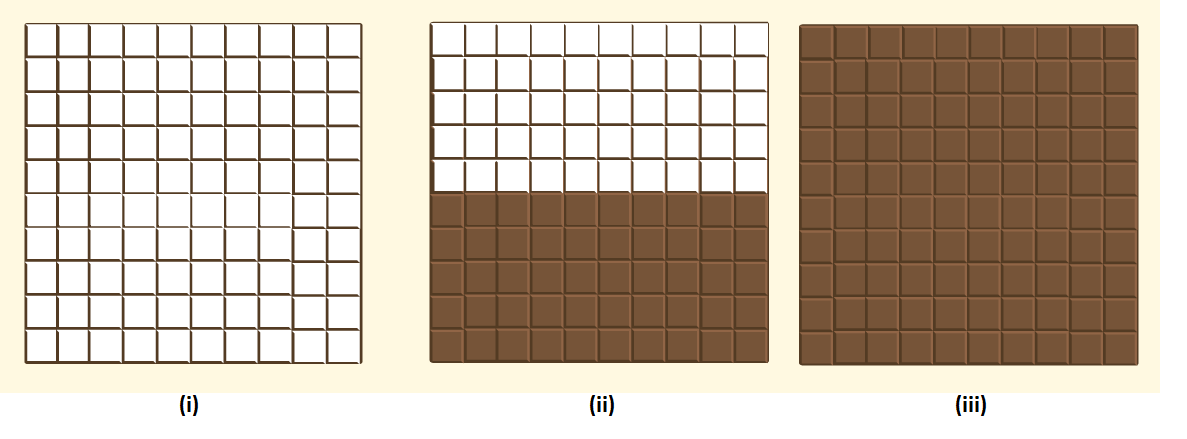# Percentage

In mathematics, a percentage is a number or ratio that can be expressed as a fraction of 100. If we have to calculate percent of a number, divide the number by the whole and multiply by 100. Hence, the percentage means, a part per hundred. The word per cent means per 100. It is represented by the symbol “%”

Examples of percentages are:

• 10% is equal to 1/10 fraction
• 20% is equivalent to ⅕ fraction
• 25% is equivalent to ¼ fraction
• 50% is equivalent to ½ fraction
• 75% is equivalent to ¾ fraction
• 90% is equivalent to 9/10 fraction

Percentages have no dimension. Hence it is called a dimensionless number. If we say, 50% of a number, then it means 50 per cent of its whole.

Percentages can also be represented in decimal or fraction form, such as 0.6%, 0.25%, etc. In academics, the marks obtained in any subject are calculated in terms of percentage. Like, Ram has got 78% of marks in his final exam. So, this percentage is calculated on account of the total marks obtained by Ram, in all subjects to the total marks.## Percentage Formula

To determine the percentage, we have to divide the value by the total value and then multiply the resultant by 100.

Percentage formula = (Value/Total value) × 100

Example: 2/5 × 100 = 0.4 × 100 = 40 per cent

## How to calculate the percentage of a number?

To calculate the percentage of a number, we need to use a different formula such as:

P% of Number = X

where X is the required percentage.

If we remove the % sign, then we need to express the above formulas as;

P/100 * Number = X

Example: Calculate 10% of 80.

Let 10% of 80 = X

10/100 * 80 = X

X = 8

## Percentage Difference Formula

If we are given with two values and we need to find the percentage difference between these two values, then it can be done using the formula:

$$\begin{array}{l}Percentage~Difference = \frac{\left|N_{1}-N_{2}\right|}{\left[\frac{\left(N_{1}+N_{2}\right)}{2}\right]} \times 100\end{array}$$

For example, if 20 and 30 are two different values, then the percentage difference between them will be:

% difference between 20 and 30 =

$$\begin{array}{l}Percentage~Difference = \frac{\left|20-30\right|}{\left[\frac{\left(20+30\right)}{2}\right]} \times 100\end{array}$$

## Percentage Increase and Decrease

The percentage increase is equal to the subtraction of the original number from a new number, divided by the original number and multiplied by 100.

% increase = [(New number – Original number)/Original number] x 100

where,

increase in number = New number – original number

Similarly, a percentage decrease is equal to the subtraction of a new number from the original number, divided by the original number and multiplied by 100.

% decrease = [(Original number – New number)/Original number] x 100

Where decrease in number = Original number – New number

So basically if the answer is negative then there is a percentage decrease.

## Solved Example

Two quantities are generally expressed on the basis of their ratios. Here, let us understand the concepts of percentage through a few examples in a much better way.

 Example: Let a bag contain 2 kg of apples and 3kg of grapes. Find the ratio of quantities present, and the percentage occupied by each. Solution: The number of apples and grapes in a bag can be compared in terms of their ratio, i.e. 2:3. The actual interpretation of percentages can be understood as follows: The same quantity can be represented in terms of the percentage occupied, which can be done as given below. Total quantity present = 5 kg Ratio of apples (in terms of total quantity) $$\begin{array}{l}=\large \frac{2}{5}\end{array}$$ = $$\begin{array}{l}\frac{2}{5} \times \frac{100}{100}\end{array}$$ From the definition of percentage, it is the ratio that is expressed per hundred, (1/100) = 1% Thus, Percentage of Apples = (2/5) × 100 = 40% Percentage of Grapes = (3/5) × 100 = 60%

## Percentage Chart

The percentage chart is given here for fractions converted into percentages.

 Fractions Percentage 1/2 50% 1/3 33.33% 1/4 25% 1/5 20% 1/6 16.66% 1/7 14.28% 1/8 12.5% 1/9 11.11% 1/10 10% 1/11 9.09% 1/12 8.33% 1/13 7.69% 1/14 7.14% 1/15 6.66%

## Converting Fractions to Percentage

A fraction can be represented by

$$\begin{array}{l}\large \frac{a}{b}\end{array}$$
.

Multiplying and dividing the fraction by 100, we have

$$\begin{array}{l} \frac{a}{b} \times \frac{100}{100}\end{array}$$

$$\begin{array}{l} =\left ( \frac{a}{b} \times 100 \right ) \frac{1}{100}\end{array}$$
………………(i)

From the definition of percentage, we have;

(1/100) = 1%

Thus, equation (i) can be written as:

(a/b) × 100%

Therefore, a fraction can be converted to a percentage simply by multiplying the given fraction by 100.

## Percentage Questions

Q.1: If 16% of 40% of a number is 8, then find the number.

Solution:

Let X be the required number.

Therefore, as per the given question,

(16/100) × (40/100) × X = 8

So, X = (8 × 100 × 100) / (16 × 40)

= 125

Q.2: What percentage of 2/7 is 1/35 ?

Solution:

Let X% of 2/7 is 1/35.

∴ [(2/7) / 100] × X = 1/35

⇒ X = (1/35) × (7/2) × 100

= 10%

Q.3: Which number is 40% less than 90?

Solution:

Required number = 60% of 90

= (90 x 60)/100

= 54

Therefore, the number 54 is 40% less than 90.

Q.4: The sum of (16% of 24.2) and (10% of 2.42) is equal to what value?

Solution:

As per the given question ,

Sum = (16% of 24.2) + (10% of 2.42)

= (24.2 × 16)/100 + (2.42 × 10)/100

= 3.872 + 0.242

= 4.114

## Word Problems

Q.1: A fruit seller had some apples. He sells 40% apples and still has 420 apples. Originally, he had how many apples?

Solution:

Let he had N apples, originally.

Now, as per the given question, we have;

(100 – 40)% of N = 420

⇒ (60/100) × N = 420

⇒ N = (420 × 100/60) = 700

Q.2: Out of two numbers, 40% of the greater number is equal to 60% of the smaller. If the sum of the numbers is 150, then the greater number is?

Solution:

Let X be the greater number.

∴ Smaller number = 150 – X {given that the sum of two numbers is 150}

According to the question,

(40 × X)/100 = 60(150 – X)/100

⇒ 2p = 3 × 150 – 3X

⇒ 5X = 3 × 150

⇒ X = 90

## Difference between Percentage and Percent

The words percentage and percent are related closely to each other.

Percent ( or symbol %) is accompanied by a specific number.

E.g., More than 75% of the participants responded with a positive response to abjure.

The percentage is represented without a number.

E.g., The percentage of the population affected by malaria is between 60% and 65%.

Fractions, Ratios, Percents and Decimals are interrelated with each other. Let us look at the conversion of one form to another:

 S.no Ratio Fraction Percent(%) Decimal 1 1:1 1/1 100 1 2 1:2 1/2 50 0.5 3 1:3 1/3 33.333 0.3333 4 1:4 1/4 25 0.25 5 1:5 1/5 20 0.20 6 1:6 1/6 16.667 0.16667 7 1:7 1/7 14.285 0.14285 8 1:8 1/8 12.5 0.125 9 1:9 1/9 11.111 0.11111 10 1:10 1/10 10 0.10 11 1:11 1/11 9.0909 0.0909 12 1:12 1/12 8.333 0.08333 13 1:13 1/13 7.692 0.07692 14 1:14 1/14 7.142 0.07142 15 1:15 1/15 6.66 0.0666

## Percentage in Maths

Every percentage problem has three possible unknowns or variables :

• Percentage
• Part
• Base

In order to solve any percentage problem, you must be able to identify these variables.

Look at the following examples. All three variables are known:

Example 1: 70% of 30 is 21

70 is the percentage.

30 is the base.

21 is the part.

Example 2: 25% of 200 is 50

25 is the percent.

200 is the base.

50 is the part.

Example 3: 6 is 50% of 12

6 is the part.

50 is the percent.

12 is the base.

## Percentage Tricks

To calculate the percentage, we can use the given below tricks.

 x % of y = y % of x

Example- Prove that 10% of 30 is equal to 30% of 10.

Solution- 10% of 30 = 3

30% of 10 = 3

Therefore, they are equal i.e. x % of y = y % of x holds true.

## Marks Percentage

Students get marks in exams, usually out of 100. The marks are calculated in terms of per cent. If a student has scored out of total marks, then we have to divide the scored marks by total marks and multiply by 100. Let us see some examples here:

 Marks obtained Out of Total Marks Percentage 30 100 (30/100)× 100 = 30% 10 20 (10/20) × 100 = 50% 23 50 (23/50) × 100 = 46% 13 40 (13/40) × 100 = 32.5% 90 120 (90/120) × 100 = 75%

## Problems and Solutions

 Question 1:- Suman has a monthly salary of $1200. She spends$280 per month on food. What percent of her monthly salary does she save? Solution: Suman’s monthly salary = $1200 Savings of Suman =$(1200 – 280) = \$ 920 Fraction of salary she saves = 920/1200 Percentage of salary she saves  = (920/1200) × 100 = 920/12% = 76.667%  Question 2:- Below given are three grids of chocolate. What percent of each White chocolate bar has a Dark chocolate bar?Solution: Each grid above has 100 white chocolate blocks. For each white chocolate bar, the ratio of the number of dark chocolate boxes to the total number of white chocolate bars can be represented as a fraction. (i) 0 dark and 100 white. i.e., 0 per 100 or 0%. (ii) 50 dark and 50 white. i.e., 50 per 100 or 50%. (iii) 100 dark and 0 white. i.e., 100 per 100 or 100%.

## Frequently Asked Questions – FAQs

### What do you mean by percentage?

In maths, a percentage is a value or ratio that shows a fraction of 100. Percent means per 100. It does not have any units.

### What is the symbol of percentage?

Percentage is denoted by ‘%’ symbol. It is also termed as per cent.

### What is the percentage formula?

The formula to calculate the percentage of a number out of another number is:
Percentage = (Original number/Another number) x 100

### What is the percentage of 45 out of 150?

(45/150) × 100 = 30%

40% of 120
= (40/100) × 120
= 48